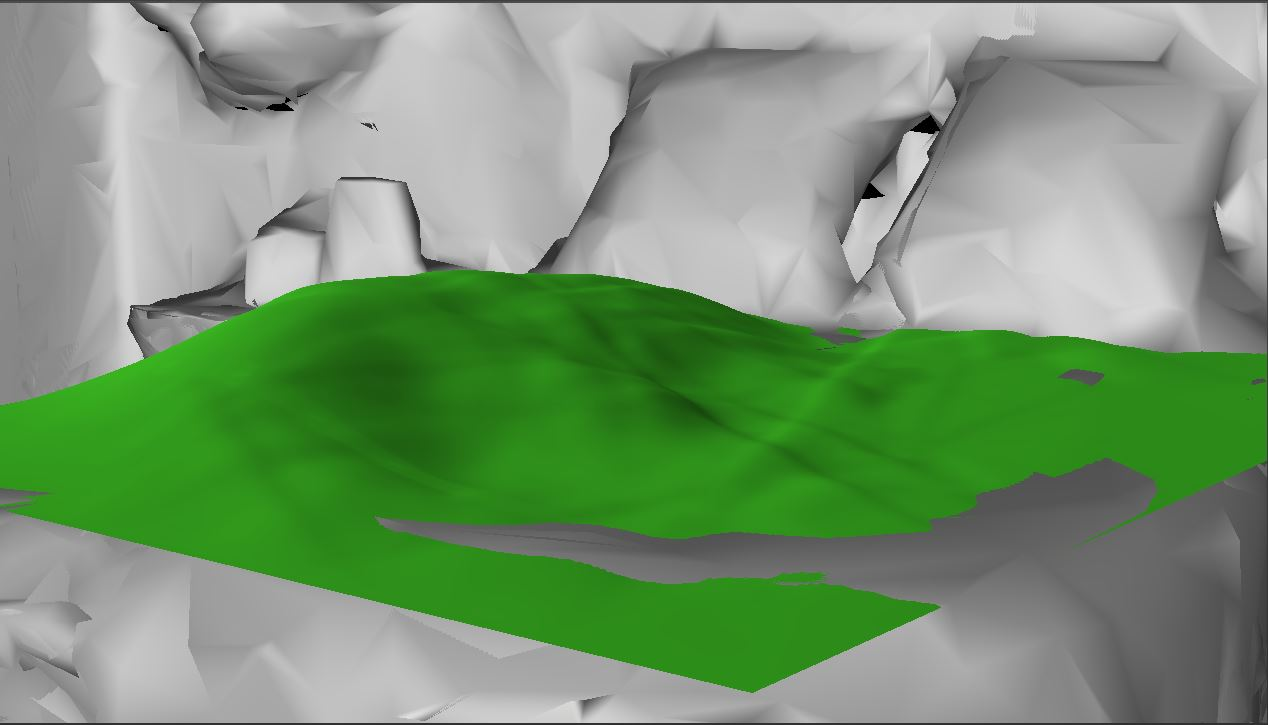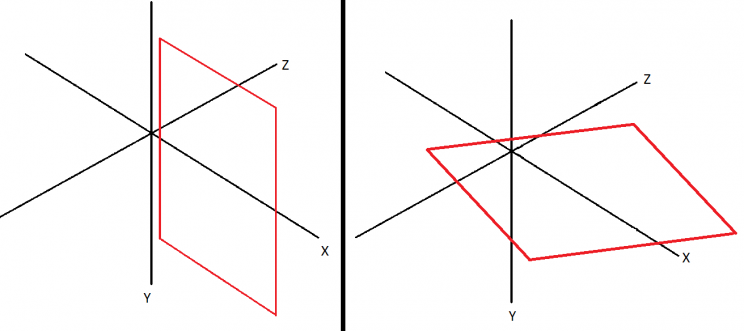# HoloLens Terrain Generation Demo Part 14 – Fixing Terrain Selection

In Part 12 I mentioned that the changes I made to the Terrain class to match location and orientation to that of the selected Surface Plane had broken the code that allows us to click/tap on the Terrain. I’m going to take this post to go over how it broke and how I fixed it.

I originally described my method for clicking on the Terrain in Part 9. The basic idea was to get the position of the camera and the direction it was facing (the gaze vector) and intersect the resulting ray with an Axis Aligned Bounding Box representing the Terrain.

```bool Terrain::CaptureInteraction(SpatialInteraction^ interaction) {
// Intersect the user's gaze with the terrain to see if this interaction is
// meant for the terrain.
auto gaze = interaction->SourceState->TryGetPointerPose(m_anchor->CoordinateSystem);

// calculate AABB for the terrain.
AABB vol;
vol.max.x = m_position.x + m_width;
vol.max.y = m_position.y + FindMaxHeight();
vol.max.z = m_position.z + m_height;
vol.min.x = m_position.x;
vol.min.y = m_position.y;
vol.min.z = m_position.z;

if (RayAABBIntersect(position, look, vol)) {
// if so, handle the interaction and return true.
m_gestureRecognizer->CaptureInteraction(interaction);
return true;
}

// if it doesn't intersect, then don't handle the interaction and return false.
return false;
}```

This worked well because the Terrain was aligned with the axes of its coordinate system and we find the gaze ray in that same coordinate system. Also, the Terrain’s position was defined relative to this coordinate system. This is no longer how we are positioning and orienting the terrain.
For all intents and purposes, our Surface Plane is defined as a Bounding Box with its center located relative to the defined coordinate system. The Bounding Box is implemented such that it assumes a vertical xy-plane and is then rotated into the correct orientation from there.On the left: initial normalized orientation of plane.
On the right: final orientation of the plane after being transformed.

Given the information we have is the center of the Bounding Box, the orientation, and the extents (which we double to get the actual dimensions), I chose to use a similar system for defining the Terrain from now on.
I had originally been defining the terrain mesh as a simple plane with the first vertex at (0, 0, 0) and extending into the xz-plane (x, 0, z). I translate the mesh vertices to their final position in the Vertex Shader such that the center of the mesh is at m_position. I didn’t need to change anything to support a new position, but supporting our new orientation information required me to instead build the mesh in the xy-plane (x, y, 0) so that I could simply apply the same orientation transform to the Terrain that was being applied to the Surface Plane.
The result of this change is that our previous CaptureInteraction() method is no longer correct because we are no longer dealing with an Axis Aligned Bounding Box. We need to convert to intersecting our gaze ray against an Oriented Bounding Box. Luckily, this is a relatively simple change. We can still use the same AABB, but with the y-axis and z-axis flipped, and provide the transformation matrix that takes us from the Terrain’s object space to world space. We can then perform nearly the same algorithm, but with the added step of transforming the gaze vector appropriately into the Terrain’s object space.
At first, I thought this would be as simple as transforming the gaze’s position and look vectors by the inverse of the same transform matrix I normally create, but I couldn’t get this to work at all. I instead used the algorithm provided here to perform the intersection test. The algorithm is nearly identical to our original RayAABBIntersect() function, except that it uses dot products between the gaze vector and each axis from the transformation matrix. I won’t reproduce the code here, but both RayAABBIntersect() and RayOBBIntersect() can be found in the MathFunctions.cpp file.
Our final CaptureInteraction() method looks like this:

```bool Terrain::CaptureInteraction(SpatialInteraction^ interaction) {
// Intersect the user's gaze with the terrain to see if this interaction is
// meant for the terrain.
auto gaze = interaction->SourceState->TryGetPointerPose(m_anchor->CoordinateSystem);

// define AABB for terrain.
MathUtil::AABB vol;
vol.min.x = vol.min.y = vol.min.z = 0.0f;
vol.max.x = m_width;
vol.max.y = m_height;
vol.max.z = FindMaxHeight();

// define transformation matrix to move AABB into world space.
XMMATRIX transform = modelTranslation * orientation;

// convert our transformation matrix to the Numerics::float4x4 format.
XMFLOAT4X4 trans;
XMStoreFloat4x4(&trans, transform);
float4x4 t;
t.m11 = trans._11;
t.m12 = trans._12;
t.m13 = trans._13;
t.m14 = trans._14;
t.m21 = trans._21;
t.m22 = trans._22;
t.m23 = trans._23;
t.m24 = trans._24;
t.m31 = trans._31;
t.m32 = trans._32;
t.m33 = trans._33;
t.m34 = trans._34;
t.m41 = trans._41;
t.m42 = trans._42;
t.m43 = trans._43;
t.m44 = trans._44;

// perform intersection test
if (MathUtil::RayOBBIntersect(position, look, vol, t)) {
// if so, handle the interaction and return true.
m_gestureRecognizer->CaptureInteraction(interaction);
return true;
}

// if it doesn't intersect, then don't handle the interaction and return false.
return false;
}```

This seems to be working pretty well now. I’m confident enough that it is right to move on. I’m thinking my next goal will be to add texturing to the Terrain. The plan is to use the DDS file type for the textures and then implement basically the same texture splatting technique I used in my Terrain Rendering project. I haven’t decided for sure on whether to use normal maps as well, and I don’t think I’ll use tri-planar mapping. We’ll see how it looks first, anyway.